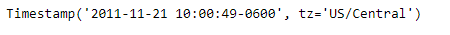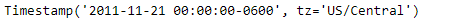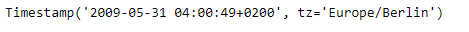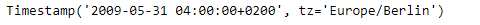Related Articles
Python | Pandas Timestamp.floor
• Last Updated : 14 Jan, 2019

Python is a great language for doing data analysis, primarily because of the fantastic ecosystem of data-centric python packages. Pandas is one of those packages and makes importing and analyzing data much easier.

Pandas` Timestamp.floor()` function return a new Timestamp floored to this resolution. The function takes the desired time series frequency as an input.

Syntax : Timestamp.floor()

Parameters :
freq : a freq string indicating the flooring resolution

Return : Timestamp

Example #1: Use `Timestamp.floor()` function to floor the given Timestamp object to Daily time series frequency.

 `# importing pandas as pd``import` `pandas as pd`` ` `# Create the Timestamp object``ts ``=` `pd.Timestamp(year ``=` `2011``,  month ``=` `11``, day ``=` `21``,``           ``hour ``=` `10``, second ``=` `49``, tz ``=` `'US/Central'``)`` ` `# Print the Timestamp object``print``(ts)`

Output :Now we will use the `Timestamp.floor()` function to floor the ts object to Daily frequency.

 `# floor the given object to daily frequency``ts.floor(freq ``=``'D'``)`

Output :As we can see in the output, the `Timestamp.floor()` function has floored the time series frequency of the given Timestamp object to the input frequency.

Example #2: Use `Timestamp.floor()` function to floor the given Timestamp object to minutely time series frequency.

 `# importing pandas as pd``import` `pandas as pd`` ` `# Create the Timestamp object``ts ``=` `pd.Timestamp(year ``=` `2009``,  month ``=` `5``, day ``=` `31``, ``       ``hour ``=` `4``, second ``=` `49``, tz ``=` `'Europe/Berlin'``)`` ` `# Print the Timestamp object``print``(ts)`

Output :Now we will use the `Timestamp.floor()` function to floor the ts object to minutely frequency.

 `# floor the given object to minutely frequency``ts.floor(freq ``=``'T'``)`

Output :As we can see in the output, the `Timestamp.floor()` function has floored the time series frequency of the given Timestamp object to the input frequency.

Attention geek! Strengthen your foundations with the Python Programming Foundation Course and learn the basics.

To begin with, your interview preparations Enhance your Data Structures concepts with the Python DS Course. And to begin with your Machine Learning Journey, join the Machine Learning – Basic Level Course

My Personal Notes arrow_drop_up